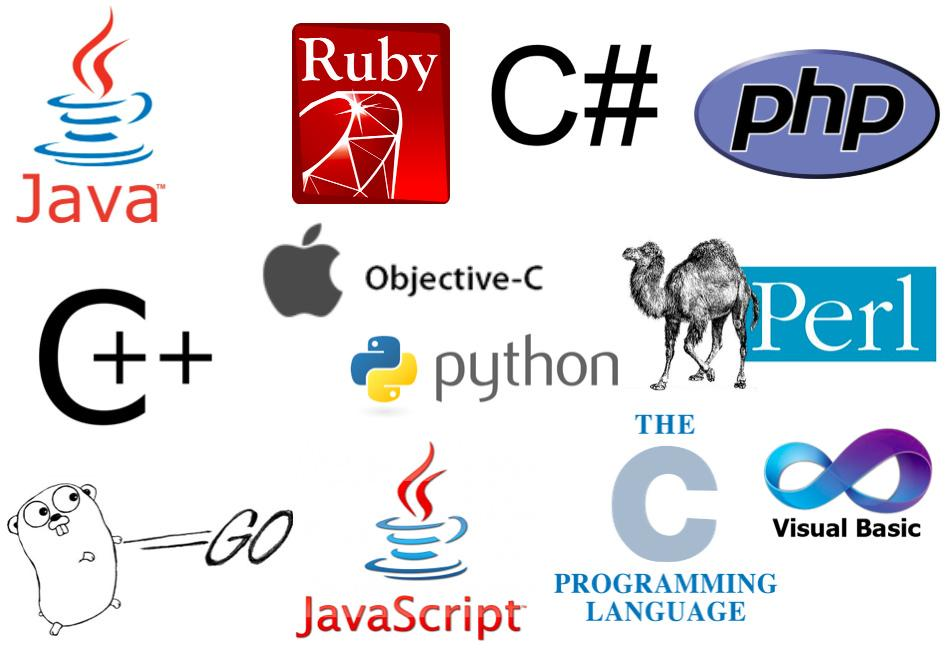“如果滑动条的当前数值大于目标数值，那么它们之间的差值应该是滑动条的当前数值减去目标数值。

``````var difference: Int
if (currentValue > targetValue) {
difference = currentValue - targetValue
} else if (targetValue > currentValue) {
difference = targetValue - currentValue
} else {
difference = 0
}``````

``````if (something is true) {
//then do this
} else if (something else is true) {
//then do that instead
} else {
//do something when neither of the above are true
}``````

``var difference: Int``

``if (currentValue > targetValue) {``

``difference = currentValue - targetValue``

``a = b - c``

``} else if (targetValue > currentValue) {``

``difference = targetValue - currentValue``

``````} else {
difference = 0
}``````

``````@IBAction func showAlert(){

var difference: Int
if currentValue > targetValue{

difference = currentValue - targetValue
}else if targetValue > currentValue{
difference = targetValue - currentValue
}else{
difference = 0
}

let mesage = "滑动条的当前数值是：\(currentValue)" +
"\n目标数值是： \(targetValue)" +
"\n两者的差值是： \(difference)"

let alert = UIAlertController(title:"Hello Messi",
message:mesage,
preferredStyle: .alert)
let action = UIAlertAction(title:"ok",style: .default,handler: nil)
alert.addAction(action)
present(alert, animated: true, completion: nil)
startNewRound()
}``````

Swift语言对空格拼写的要求非常严格，所以在变量和操作符（比如+）之间必须添加空格，否则你的代码里面会大量提示出错~